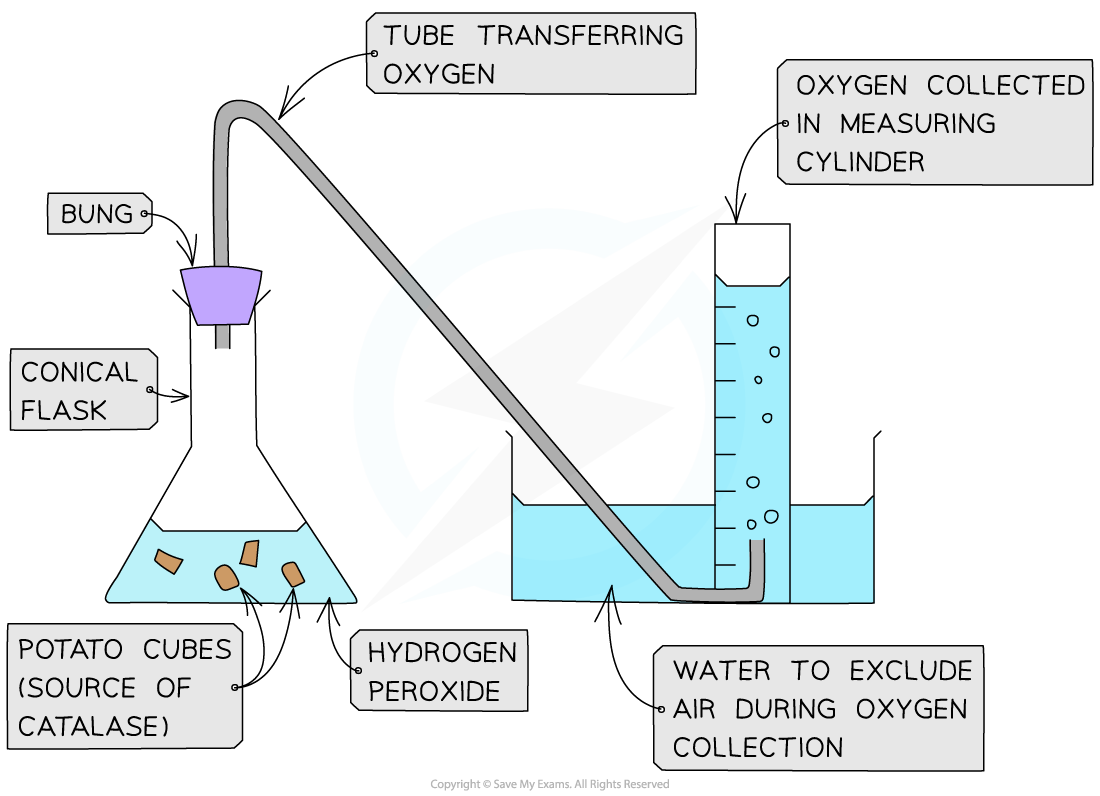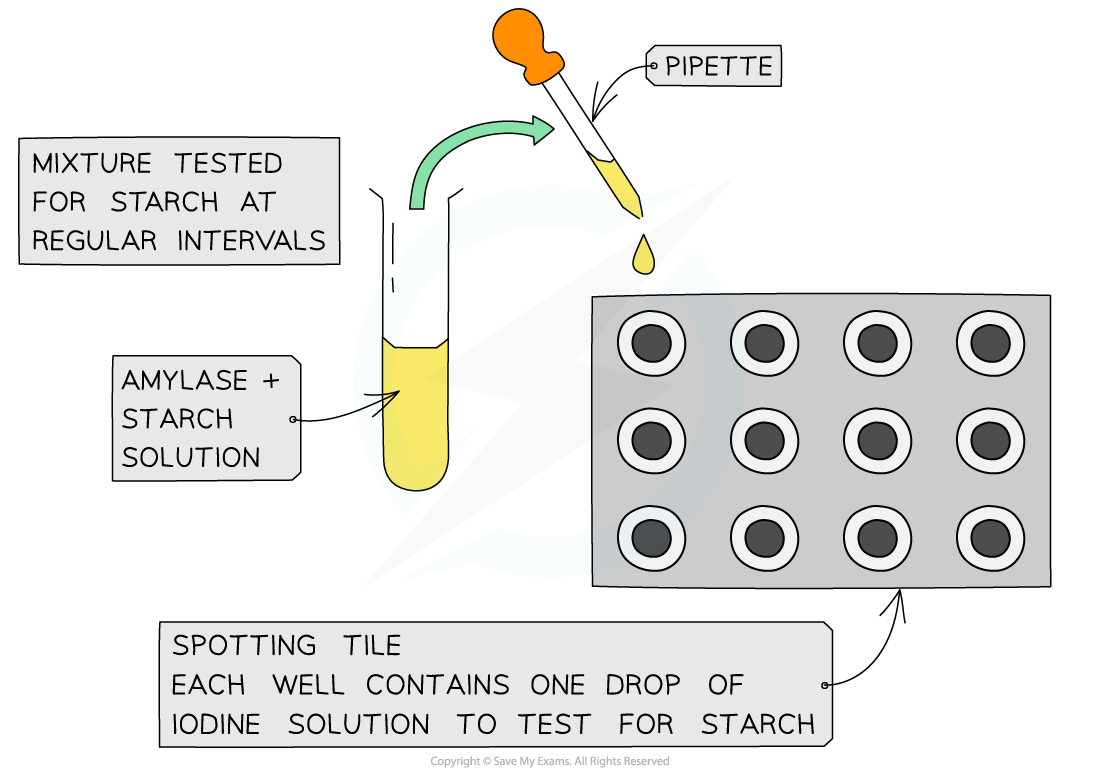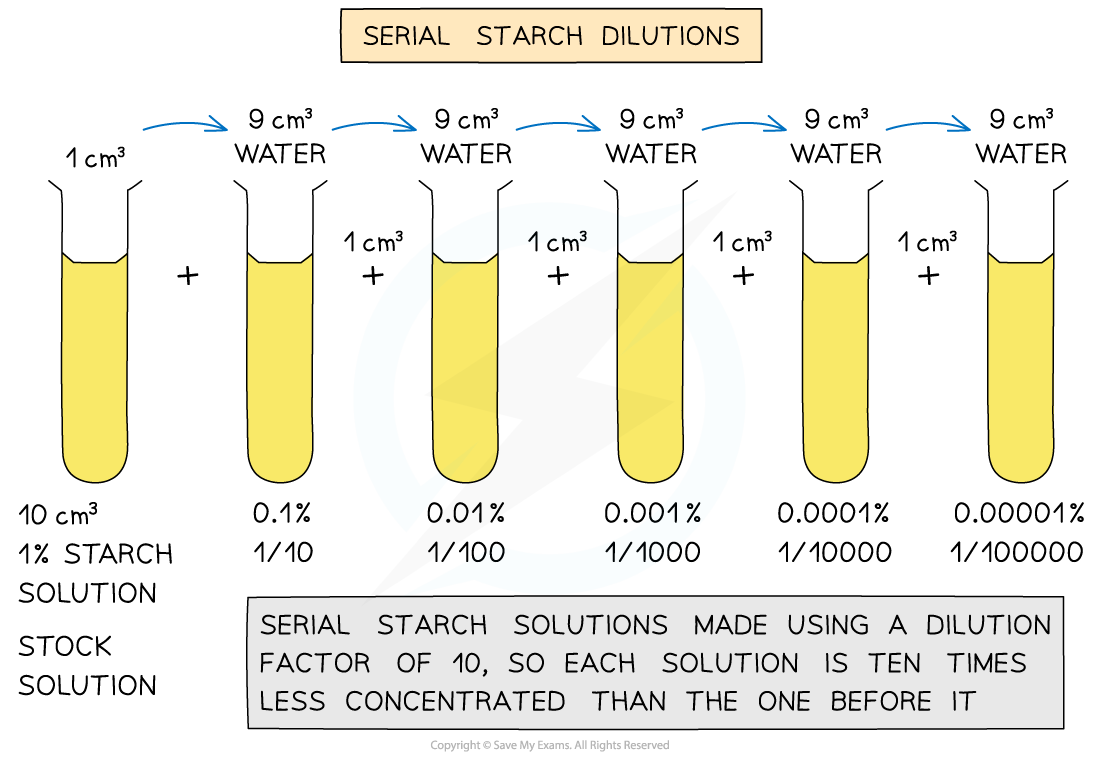# AQA A Level Biology复习笔记1.4.4 Required Practical: Measuring Enzyme Activity

### Required Practical: Measuring Enzyme Activity

• The progress of enzyme-catalysed reactions can be investigated by:
• Measuring the rate of formation of a product using catalase
• Measuring the rate of disappearance of a substrate using amylase

#### Investigating catalase activity

• In this investigation, the rate of product formation is used to measure the rate of an enzyme-controlled reaction:
• Hydrogen peroxide is a common but toxic by-product of metabolism
• This means it must be broken down quickly
• Catalase is an enzyme found in the cells of most organisms that breaks down hydrogen peroxide into water and oxygen
• Hydrogen peroxide and catalase are combined and the volume of oxygen generated is measured in a set time
• The rate of reaction can then be calculatedExperimental set-up for investigating the rate of formation of a product using catalase

#### Investigating amylase activity using iodine

• In this investigation, the rate of substrate disappearance is used to compare rates of reaction under different conditions
• Amylase is a digestive enzyme that hydrolyses starch into maltose and glucose
• Amylase functions best at pH 7 and 37oC (all enzymes operate best under specific conditions)
• Amylase and starch are combined and this reaction mixture is then tested for starch at regular time intervals
• This can be done by taking samples from the reaction mixture at each time interval and adding each sample to some iodine in potassium iodide solution
• Starch forms a blue-black colour with this solution
• If no starch is present, the iodine solution remains yellow-brown

• In this way, the time taken for starch to be broken down can be measured
• The investigation can be repeated under a variety of conditions (eg. by altering pH, temperature, enzyme concentration or starch concentration) and the reaction rates can then be comparedExperimental set-up for investigating the rate of disappearance of a substrate using amylase

#### Investigating the effect of starch concentration on amylase activity using colourimetry

• A colourimeter is able to measure light absorbance (how much light is absorbed) or light transmission (how much light passes through) a substance
• Colourimetry can be used in any enzyme-catalysed reaction that involves colour change
• As the colour breaks down the transmission increases or light absorption decreases and this can be used to measure the rate of the reaction
• For example, a colourimeter can be used to follow the progress of a starch-amylase catalysed reaction as the amylase breaks the starch down into maltose
• This can be carried out as follows:
• Colourimeter calibration: this is an important step in a colourimetric investigation and in this case a weak iodine solution can be used to calibrate the colourimeter as the end point (or 100% transmission)
• Preparation of a starch solution of known concentration (stock solution), from which a range of concentrations are made using serial dilutions (method outlined in diagram below)
• Following calibration and switching on the red filter (to maximise the percentage transmission or absorbance), the colourimeter is used to measure the percentage absorbance or percentage transmission values
• Sometimes a reagent or indicator is used to produce the colours detected by the colourimeter and sometimes the solutions themselves absorb light waves
• A calibration graph is then plotted of starch concentration (X-axis) vs percentage absorbance or percentage transmission (Y-axis)Serial dilution of starch to make a range of concentrations